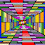## Tuesday, June 24, 2014

### 33 | Cordell Hull, Father of the United Nations

Cordell Hull is best remembered for two accomplishments; first he is the longest serving Secretary of States under FDR's Presidency from the years 1933-1944, for a total of 11-years.  Please notice the years '33 and '44.  Cordell was the 47th Secretary of State.  Cordell Hull is also widely recognized as the founder of the United Nations, a symbol that is made up of "33-spaces".  Let's see what these "33-spaces" have in common with the name Cordell.
• Cordell = 3+6+9+4+5+3+3 = 33
• Hull = 8+3+3+3 = 17
Before joining the FDR administration as Secretary of State, Hull attended Cumberland Law School in Lebanon, Tennessee, the nation's eleventh oldest law school.  To date, Cordell Hull is the only alumni of that school to win the Nobel Peace Prize.  Cumberland was established July of 1847.  Again, notice the year '47, much like '4th of July'...  There is also a connection between Cumberland and the United Nations.
• Cumberland = 3+3+4+2+5+9+3+1+5+4 = 39
• The United Nations is in NYC
• It is 39-floors tall
• New York = 5+5+5+7+6+9+2 = 39
• NY = 39
• 7/26/1788 = 7+2+6+1+7+8+8 = 39
• *York = 7+6+9+11 = 33
Cordell Hull was born October 2, 1871, and died July 23, 1955.  Something tells me his death in '55, the same year Einstein and many other notable figures died, was not by natural causes.  It should also be noted that his birth month, October, is the only month with "33-name numerology".
• October = 6+3+2+6+2+5+9 = 33
This "33" in names thing, it predates the English language.  Proof?  Let us examine the word "name" it self in numerology terms.
• Name = 14+1+13+5 = 33
• Code Name = 3+6+4+5+5+1+4+5 = 33
It goes beyond names however, it also include dates.  The Charter for the United Nations was signed on June 26, 1945; emphasis on the twenty-sixth day.
• 6/26/1945 = 6+2+6+1+9+4+5 = 33
To close, let us examine the United Nations symbol, and count all "33 spaces".  To get "33", make sure you count the middle space too.

1.2.